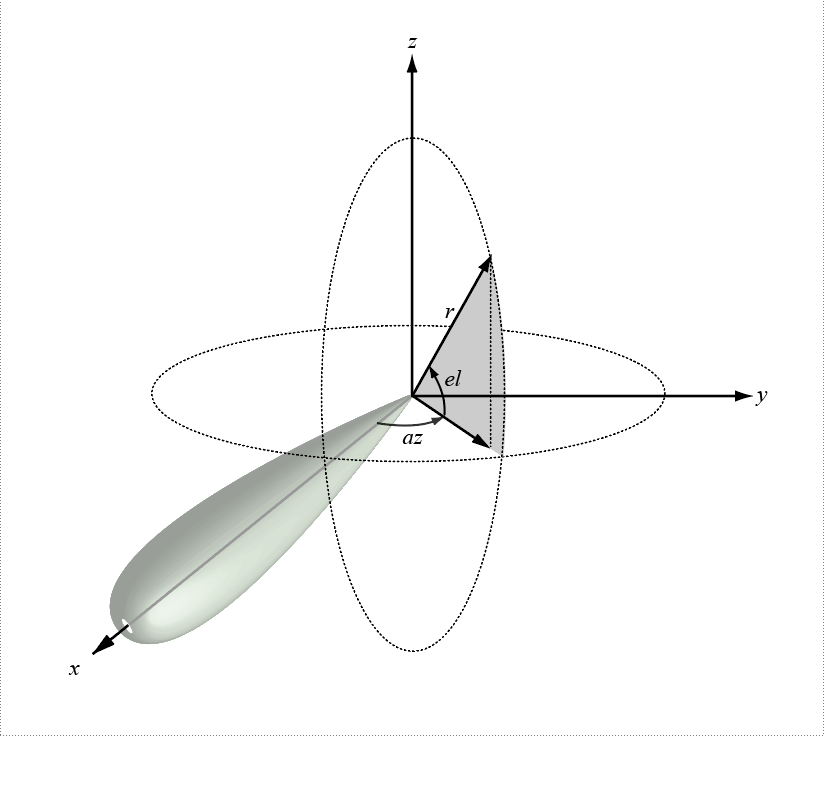# global2localcoord

Convert global to local coordinates

## Syntax

```lclCoord = global2localcoord(gCoord, OPTION) gCoord = global2localcoord(___,localOrigin) gCoord = global2localcoord(___,localAxes) ```

## Description

`lclCoord = global2localcoord(gCoord, OPTION)` converts global coordinates `gCoord` to local coordinates `lclCoord`. `OPTION` determines the type of global-to-local coordinate transformation. In this syntax, the global coordinate origin is located at (0,0,0) and the coordinate axes are the unit vectors in the x, y, and z directions.

`gCoord = global2localcoord(___,localOrigin)` specifies the origin of the local coordinate system, `localOrigin`.

`gCoord = global2localcoord(___,localAxes)` specifies the axes of the local coordinate system, `localAxes`.

## Input Arguments

`gCoord`

Global coordinates in rectangular or spherical coordinate, specified as a 3-by-N matrix. Each column represents one set of global coordinates.

If the coordinates are in rectangular form, each column contains the (x,y,z) components. Units are in meters.

If the coordinates are in spherical form, each column contains (az,el,r) components. az is the azimuth angle in degrees, el is the elevation angle in degrees, and r is the radius in meters.

The origin of the global coordinate system is assumed to be located at (0, 0, 0). The global system axes are the standard unit basis vectors in three-dimensional space, (1, 0, 0), (0, 1, 0), and (0, 0, 1).

`OPTION`

Type of coordinate transformation, specified as a character vector. Valid types are

OPTIONTransformation
`'rr'`Global rectangular to local rectangular
`'rs'`Global rectangular to local spherical
`'sr'`Global spherical to local rectangular
`'ss'`Global spherical to local spherical

`localOrigin`

Origin of local coordinate system, specified as a 3-by-N matrix containing the rectangular coordinates of the local coordinate system origin with respect to the global coordinate system. N must match the number of columns of `gCoord`. Each column represents a separate origin. However, you can specify `localOrigin` as a 3-by-1 vector. In this case, `localOrigin` is expanded into a 3-by-N matrix with identical columns.

Default: `[0;0;0]`

`localAxes`

Axes of local coordinate system, specified as a 3-by-3-by-N array. Each page contains a 3-by-3 matrix representing a different local coordinate system axes. The columns of the 3-by-3 matrices specify the local x, y, and z axes in rectangular form with respect to the global coordinate system. However, you can specify `localAxes` as a single 3-by-3 matrix. In this case, `localAxes` is expanded into a 3-by-3-by-N array with identical 3-by-3 matrices. The default is the identity matrix.

Default: `[1 0 0;0 1 0;0 0 1]`

## Output Arguments

 `lclCoord` Local coordinates in rectangular or spherical coordinate form, returned as a 3-by-N matrix. The dimensions of `lclCoord` match the dimensions of `gCoord`.

## Examples

collapse all

Convert global rectangular coordinates, (0,1,0), to local rectangular coordinates. The local coordinate origin is (1,1,1).

`lclCoord = global2localcoord([0;1;0],'rr',[1;1;1])`
```lclCoord = 3×1 -1 0 -1 ```

Convert global spherical coordinates to local rectangular coordinates.

`lclCoord = global2localcoord([45;45;50],'sr',[50;50;50])`
```lclCoord = 3×1 -25.0000 -25.0000 -14.6447 ```

collapse all

### Azimuth and Elevation Angles

The azimuth angle of a vector is the angle between the x-axis and the orthogonal projection of the vector onto the xy plane. The angle is positive in going from the x axis toward the y axis. Azimuth angles lie between –180 and 180 degrees. The elevation angle is the angle between the vector and its orthogonal projection onto the xy-plane. The angle is positive when going toward the positive z-axis from the xy plane. By default, the boresight direction of an element or array is aligned with the positive x-axis. The boresight direction is the direction of the main lobe of an element or array.

Note

The elevation angle is sometimes defined in the literature as the angle a vector makes with the positive z-axis. The MATLAB® and Communications Toolbox™ products do not use this definition.

This figure illustrates the azimuth and elevation angles of a direction vector.## References

 Foley, J. D., A. van Dam, S. K. Feiner, and J. F. Hughes. Computer Graphics: Principles and Practice in C, 2nd Ed. Reading, MA: Addison-Wesley, 1995.